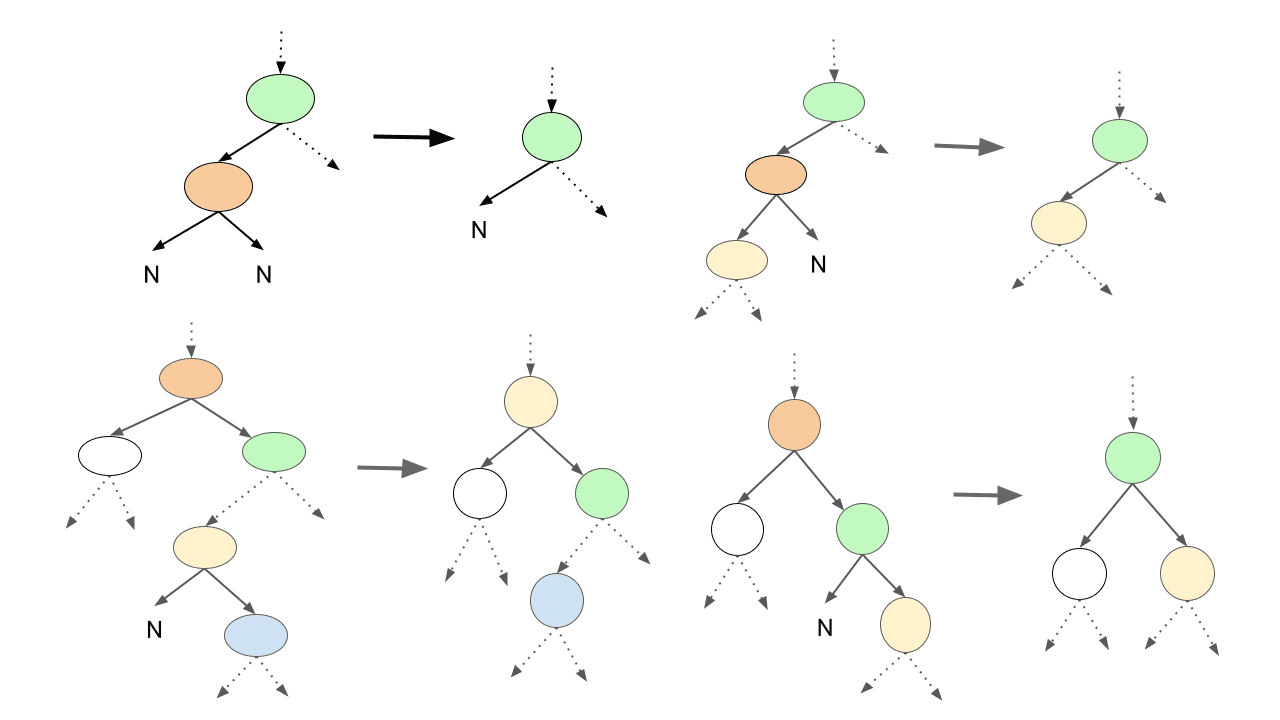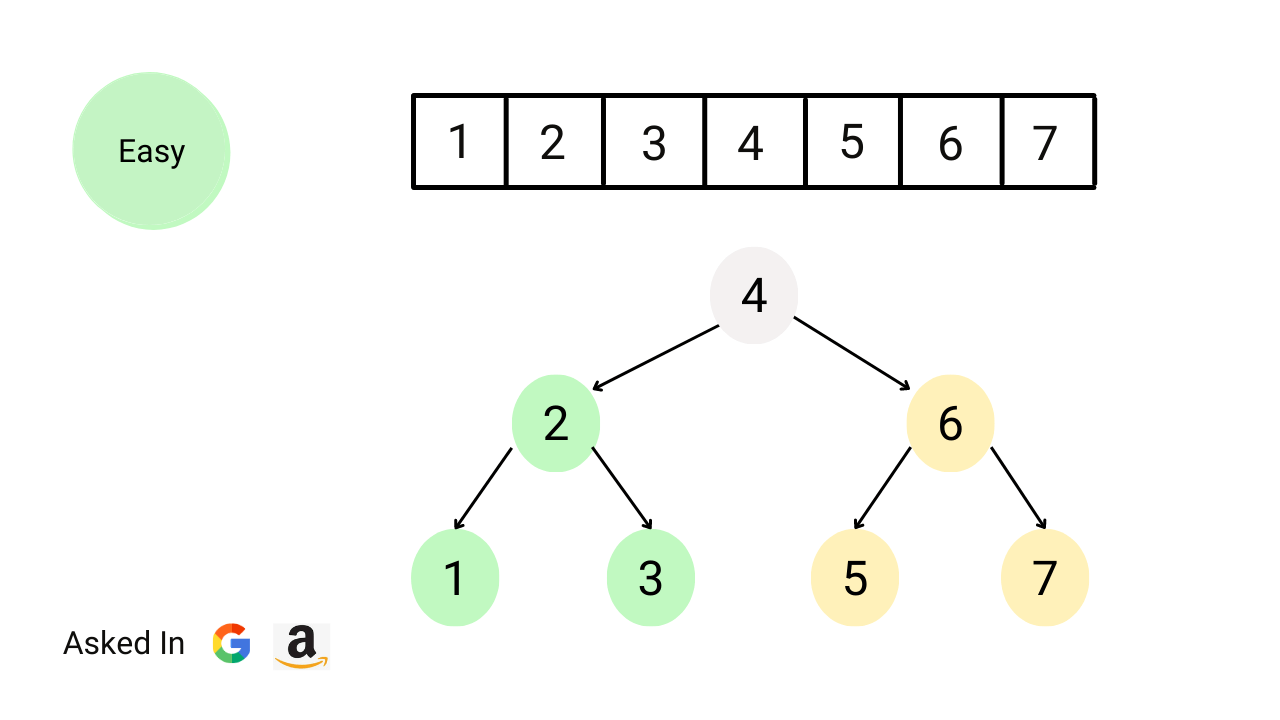# binary-treeDelete Node in a Binary Search Tree

Given a binary search tree and a key value. Write a program to delete the given key from the binary search tree and return the updated root node. This is an excellent problem to learn pointer manipulation in binary trees and problem-solving using both iterative and recursive approaches.Convert sorted array to balanced BST

Write a program to convert a sorted array of integers into a balanced binary search tree. Each node in the tree must follow the BST property, and the height of the left and right subtrees should be as close to equal as possible. Note: This is an excellent problem to learn problem-solving using the divide and conquer approach.Merge Two Binary Trees

Given two binary trees, write a program to merge them into a single binary tree. We need to merge them such that if two nodes overlap then add them and put them into the new tree at the same position. Otherwise, put the non-NULL node in the new tree. Note: This is an excellent problem to learn problem solving using iterative and recursive preorder traversal.Fenwick Tree (Binary Indexed Tree)

A Fenwick tree, also known as a binary indexed tree (BIT), is a data structure that allows for efficient updates and prefix sum calculations on an array. It has a time complexity of O(logn) for both updates and range sum queries. Fenwick trees were first described in a 1994 paper by Peter M. Fenwick titled "A new data structure for cumulative frequency tables.Level Order Traversal (BFS Traversal) of Binary Tree

Level order traversal accesses nodes in level by level order. This is also called breadth first search or BFS traversal. Here we start processing from the root node, then process all nodes at the first level, then process all nodes at the second level, and so on. In other words, we explore all nodes at the current level before going to nodes at the next level.Height (Maximum Depth) of a Binary Tree

Given a binary tree, write a program to find its height. In other words, we are given a binary tree and we need to calculate the maximum depth of the binary tree. The height or maximum depth of a binary tree is the total number of edges on the longest path from the root node to the leaf node. Note: This is an excellent problem to learn problem-solving using DFS and BFS traversal.Minimum Absolute Difference in BST

Given the root of a binary search tree (BST), write a program to find the absolute minimum difference between the values of any two nodes in the tree. Here node values in the tree can be positive, negative, or zero. Note: This is an excellent problem to learn problem-solving using iterative and recursive inorder traversal of a binary tree.Kth Largest Element in BST

Given the root of a BST and an integer k, write a program to find the kth largest value among all the nodes' values in the binary search tree. Note: This is an excellent problem to learn problem solving using recursive and iterative inorder traversal and data structure augmentation (storing extra information inside BST nodes for solving a problem).Iterative Preorder, Inorder and Postorder Traversal Using Stack

We can easily implement recursive binary tree traversals (preorder, inorder, and postorder) iteratively using a stack. We need to understand the flow of recursive calls in DFS traversal and mimic what the compiler does in the background. So, we need to follow a similar process and use our own stack to simulate the recursive binary tree traversal using iteration or without using recursion.Preorder, Inorder and Postorder Traversal using Recursion

There are three types of recursive tree traversals: preorder, inorder and postorder. This classification is based on the visit sequence of root node 1) Preorder traversal: root is visited first 2) Inorder traversal: root is visited after left subtree 3) Postorder traversal: root is visited last. These are also called depth first search or DFS traversal.AVL Tree

AVL Trees is a height-balanced BST named after its inventors, Adelson-Velsky and Landis. This is a variation of binary search trees, also known as self-balancing BST. The property of AVL Trees: The absolute difference of height between left and right subtree is at max 1, which could help us to perform bst operations in O(logn) time complexity.What is Heap Data Structure? Properties and Applications

Heap is a complete binary tree structure where each node satisfies a heap property. We learn two types of heap data structure: 1) Max heap, which satisfies the max heap property, and 2) Min heap, which satisfies the min-heap property. We use heap data structures to implement priority queues and solve several coding problems efficiently.Introduction to Binary Search Tree (BST) in Data Structure

Binary search tree (BST) is a sorted binary tree, where key stored in each node must satisfy the binary search tree property: 1) Keys in the left subtree ≤ Node key 2) Keys in the right subtree ≥ Node key 3) Both subtrees must be binary search trees. In this blog, we have discussed the key properties, operations and differences of BST with a hash table.Insertion in Binary Search Tree (BST)

The root of the binary search tree and a key k is given. Write a program to insert key k into the binary search tree. Note: BST structure will change after the insertion. So we need to perform insertion in such a way that the BST property continues to hold. In this blog, we have discussed recursive and iterative implementations of insertion in BST.Searching in Binary Search Tree (BST)

We start searching from root node and traverse a path downward in binary search tree. For each node in the path, compare target key k with the node key. If equal, search is successful. If k < node key, go to the left subtree. Similarly, if k > node key, go to the right subtree. In this blog, we have discussed recursive and iterative implementations of searching in BST.Validate Binary Search Tree (BST)

Given the root of a binary tree, write a program to check whether tree is a valid binary search tree (BST) or not. To check valid bst, we verify bst property at each node: All node values in the left subtree are less than node’s value, all node values in the right subtree are greater than node’s value, and both subtrees are also binary search trees.N-ary Tree

We know that a binary tree is a rooted tree in which each node has no more than two children. We can extend this definition to an n-ary tree. If a tree is rooted in which each node has no more than n children, it is called an n-ary tree. In other words, n ary trees are tree data structures with up to n children nodes for each node present in the tree.Suffix Tree Data Structure

Suffix trees are a compressed version of the trie that includes all of a string's suffixes. It can be used to solve many string problems that occur in text editing, free-text searches, etc. Some popular applications of suffix trees are string search, finding the longest repeated substring, finding the longest common substring, data compression, etc.Binary Tree Introduction, Properties, Types and Applications

Binary tree is one of the simplest tree data structures where each node has at most two child nodes. In other words, a node in a binary tree can have 0 or 1 or 2 child nodes. In this blog, we have discussed: 1) Key terminologies 2) Types of binary tree 3) Properties of binary tree 4) Linked and array representation 5) Binary tree applications.Segment Trees (Point and Range Update, Lazy Propagation)

In this blog, we will learn how to use segment trees for efficient point and range updates. For point update query, update(idx, val), we need to increment element at index idx from the original array by val, i.e. arr[idx] = arr[idx] + val. For range update query, update(l, r, val), we increment all elements from index l to r from the original array by val.Segment Tree (Build and Range Query Operations)

A segment tree is a binary tree data structure such that each node stores information about a range. We use segment trees to efficiently answer multiple range queries on an array like range minimum, range maximum, range sum, etc. Also, it allows us to modify the array by replacing an element or an entire range of elements in logarithmic time.Left View of Binary Tree

Given a root node of a binary tree, write a program to find the left view of the given binary tree. The left view of a binary tree is a group of nodes visible when viewed from the left side. In other words, the nodes in the left view will be the first node of each level. This is an excellent problem to learn problem-solving using DFS and BFS traversal.Minimum Depth of Binary Tree

Given a binary tree, find its minimum depth. The min depth of a binary tree is the number of nodes along the shortest path from root node down to the nearest leaf node. The path has to end on a leaf node. Note: An excellent problem to understand efficient problem solving using breadth-first search (BFS) when the solution node is nearest to the root node.